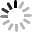### Tag Cloud

stone

Phone Number : 008615195010186

## NC/CNC MACHINING CALCULATING FORMULA

September 3, 2019

In NC machining or CNC machining working, we always met the different drawings which marked with different machining standard. That require worker to calculate dimensions with different calculation formula.

## International Standards

### MACHINING CALCULATING FORMULA FOR CALCULATING THE BORE DIAMETER OF THE TAPPING OF EXTRUSION WIRE:

Formula: External diameter of teeth – 1/2 *pitch

Example 1: Formula: M3 * 0.5 = 3 – (1/2 * 0.5) = 2.75mm

M6 x 1.0 = 6 – (1/2 x 1.0) = 5.5 mm

Example 2: Formula: M3 * 0.5 = 3 – (0.5/2) = 2.75 mm

M6*1.0=6-(1.0/2)=5.5 mm.

### MACHINING CALCULATING FORMULA FOR ENGLISH TAPPING:

1 inch = 25.4mm

Example 1: (1/4-30)

1/4 x 25.4 = 6.35 (Pitch Diameter )

25.4/30 = 0.846 (pitch)

The conversion from 1/4-30 to metric screw should be M6.35 * 0.846

Example 2: (3/16-32)

3/16*25.4=4.76 ( Pitch Diameter )

25.4/32 = 0.79 (pitch)

The conversion from 3/16-32 to metric screw should be M4.76 *0.79

Molecular/Denominator x 25.4 =Pitch diameter

Example 1: (3/8-24)

3/8 x 25.4 = 9.525 ( Pitch Diameter )

25.4 24 = 1.058 ( METRIC THREAD )

The conversion from 3/8-24 to metric thread should be M9.525 * 1.058CNC machining part

Example: 6-32

6-32 (0.06+0.013)/Code *6=0.138

0.138 * 25.4 = 3.505( Pitch Diameter )

25.4/32 = 0.635 (pitch)

The conversion of 6-32 to metric thread should be M3.505 * 0.635

#### 1.FORMULA FOR CALCULATING BORE DIAMETER:

External thread diameter- 1/2 * pitch should be:

M3.505-1/2*0.635=3.19

So the inner hole dia. of 6-32 should be 3.19

#### 2.INNER HOLE TAPPING ALGORITHMS FOR EXTRUSION WIRE:

Calculating Lower hole diameter Formula 1:

External pitch diameter – (pitch * 0.4250.475) / code = lower hole diameter

Example 1: M6 *1.0

M6-(1.0 x 0.425) = 5.575 (maximum lower hole diameter)

M6-(1.0 x 0.475)= 5.525 (minimum lower hole diameter)

Example 2: Simple formulas for calculating lower hole diameter of tapped holes by cutting wire:

M6-(1.0 x 0.85) = 5.15 (maximum)

M6-(1.0 x 0.95)=5.05 (minimum)

M6-(pitch * 0.860.96)/Code = Lower hole diameter

Example 3: M6*1.0=6-1.0=5.0+0.05=5.05

## Machining Calculating Formula for the External Diameter of Pressure Thread:

Diameter – 0.01 *0.645 *Pitch Distance

Example 1: M3 * 0.5 = 3-0.01*0.645 * 0.5 = 2.58 (external diameter)

Example 2: M6*1.0=6-0.1*0.645*1.0=5.25 (external diameter)

## Formula for calculating roll diameter of metric thread: (full teeth)

Example 1: M3 x 0.5 = 3-0.6495 x 0.5 = 2.68 (outside diameter before turning)

Example 2: M6*1.0=6-0.6495*1.0=5.35 (outside diameter before turning)

## External Diameter Depth (External Diameter) of Embossing

Outer diameter/25.4 * embossing pitch = outer diameter before embossing

Example: 4.1/25.4 x 0.8 (embossing pitch spacing) = 0.13 embossing depth should be 0.13.

## Diagonal Conversion Formula for Polygonal Materials:

Formula 1:

Hexagonal: diagonal diameter * 1.1547 = diagonal diameter

Formula 2:

## Tool Thickness (Cutter):

material outer diameter/10+0.7 Reference value

## Formula for calculating taper:

Total Length of Taper) = Degrees

Equal to the value of a triangular function

Formula 2: